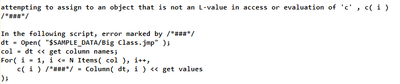Choose Language Hide Translation Bar
Highlighted

## How to allocate variables based on the number of iterations in a for loop?

Hi,

I am trying to allocate variables based on the number of iterations in a for loop.

An better explanation regarding my question is as follows:

This is my current code:

dt = Open( "\$SAMPLE_DATA/Big Class.jmp" );

col = dt<<get column names;

for (i=1, i<=nitems(col), i++,

c(i)= column(dt,i)<<get values;

);

The error message that is shown in the log is :I wanted to get all the column values for all the columns available in the same data assigned to unique variables.

Eg: All values under the first column are assigned to c1(where i=1), column 2 values are assigned to c2(where i=2), etc

If I change c(i)= column(dt,i)<<get values; --> c1= column(dt,i)<<get values; the script works fine without error.

However this is not what I am looking for since I want each variable name for each column values to be unique and is allocated baased on the number of iterations in the for loop. Can anyone help me to fix my code?

Thank you.

Best Rgds,

Val

1 ACCEPTED SOLUTION

Accepted Solutions
Highlighted

## Re: How to allocate variables based on the number of iterations in a for loop?

There are several ways to do dynamic assignments. I think the most straightforward is to build the expression as a string and then parse and evaluate it.

dt = Open("\$SAMPLE_DATA/Big Class.jmp");

col = dt << get column names;

For(i = 1, i <= N Items(col), i++,

c = "c" || Char(i);

Eval(Parse(Eval Insert("^c^= column(dt,i)<<get values")));

);

Show(c1, c2);

4 REPLIES 4
Highlighted

## Re: How to allocate variables based on the number of iterations in a for loop?

I am a little confused on what the intent is for moving the data from a data table into a structure such as you are describing, and knowing that might help in providing a solution.

There are a couple of items that might be what you are looking for.

1. Move the data into memory variables called C1, C2, etc.

This can be accomplished by replacing the line:

c( 1 ) = column(dt,i)<<get values;

with

Eval(substitute(expr( __var__  = column(dt,i)<<get values; ), expr(__var__), Parse("c" || char( i ) ) );

2. All numeric columns can be moved into a matrix, where "C[0,1]" would reference column 1, "C[0,2]" references column 2, etc.

names default to here(1);

dt=current data table();

c=dt<<get as matrix;

3. You could also move the column values into a List of Lists, where the first list in the overall structure would be the data from column 1, the second list would be column 2, etc.

names default to here(1);

dt=current data table();

C = {};

For(i=1,i<= n col(dt),i++,

c=as list(column(dt,i)<<get values)

);

Jim
Highlighted

## Re: How to allocate variables based on the number of iterations in a for loop?

Hi Jim,

Thank you for your assistance. Solutions 1 and 3 are perfect for my project.

I am actually working on multiple large data sets that varies in terms of columns count and content. Since the number of columns are different for different data sets, it is not a good idea to hard code / fix the number of variables and the names corresponding to the hard-coded variables. This is why I am looking for a dynamic solution to name my variables so that I can tell exactly which variable is tied to which column and its respective contents.

Best Regards,

Val

Highlighted

## Re: How to allocate variables based on the number of iterations in a for loop?

There are several ways to do dynamic assignments. I think the most straightforward is to build the expression as a string and then parse and evaluate it.

dt = Open("\$SAMPLE_DATA/Big Class.jmp");

col = dt << get column names;

For(i = 1, i <= N Items(col), i++,

c = "c" || Char(i);

Eval(Parse(Eval Insert("^c^= column(dt,i)<<get values")));

);

Show(c1, c2);

Highlighted

## Re: How to allocate variables based on the number of iterations in a for loop?

Hi MS,

Thank you! Yes, this is exactly what I am looking for.

Best Regards,

Val

Article Labels

There are no labels assigned to this post.